/ post--ctc Public

# Peer Review Report 1 -- Anonymous#13

Closed
opened this issue Nov 13, 2017 · 1 comment
Closed

# Peer Review Report 1 -- Anonymous #13

opened this issue Nov 13, 2017 · 1 comment
Labels

### colah commented Nov 13, 2017

 The following peer review was solicited as part of the Distill review process. Some points in this review were clarified by an editor after consulting the reviewer. The reviewer chose to keep keep anonymity. Distill offers reviewers a choice between anonymous review and offering reviews under their name. Non-anonymous review allows reviewers to get credit for the service them offer to the community. Distill is grateful to the reviewer for taking the time to review this article. Conflicts of Interest: Reviewer disclosed a minor potential conflict of interest that the editors did not view as a substantive concern. In general, this is a well written and clear overview of CTC. Even for someone who has worked with it a lot, it still helped it feel a bit clearer, especially with the dynamic programming and beam search. The biggest thing that could use some help is clarifying the formal definition of the alignments A, and how you get p(a|x) i.e. that’s where the function approximator goes.nit: this looks a little like X_s Y_s, maybe a better way of wording this.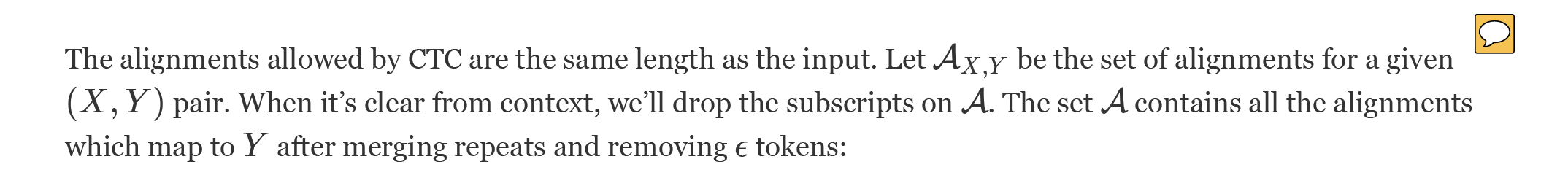Maybe clarify here what an alignment means formally. How does p(a|X) relate to p(y|X) ?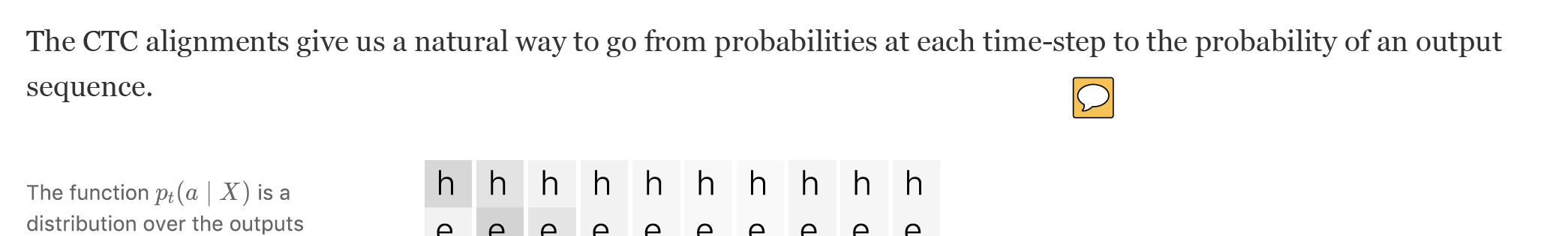By here it can be extra helpful to clarify where p(a|X) comes from. Maybe talk about p(y|X) coming from a neural network and show audio (X) and P(y|x) as first parts of this diagram?)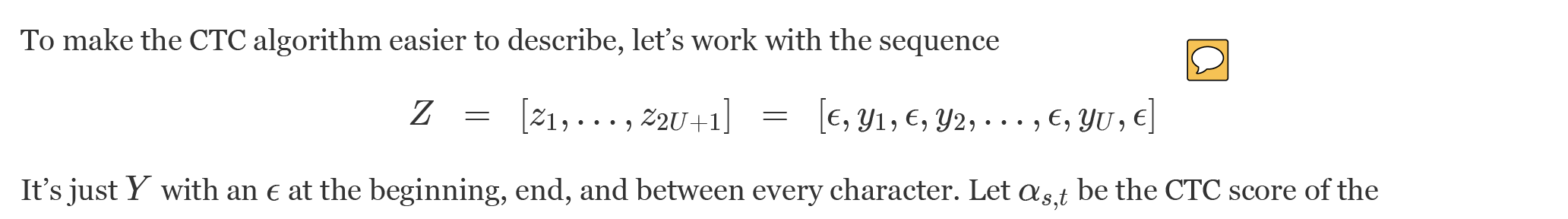Again, clarifying how to go from p(y|X) to p(z|X) might help. Also, defining the CTC score as it’s relationship to P(Y|X) can be helpful so the reader doesn’t have to wait to find out.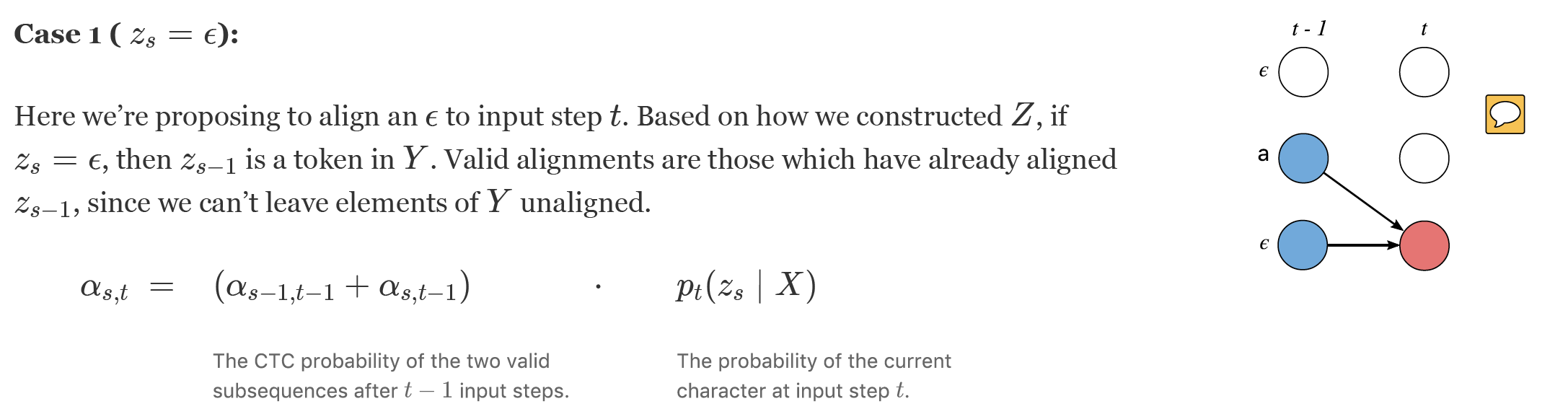These diagrams are great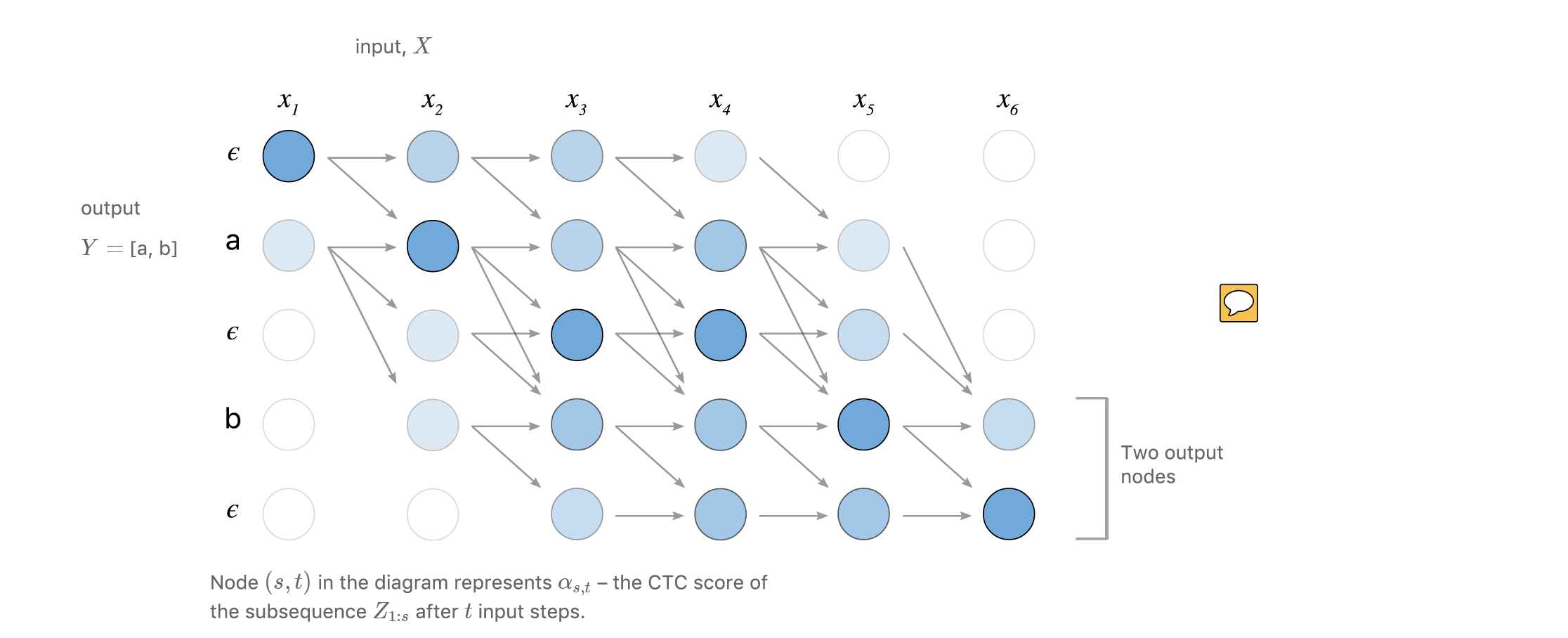The diagrams above were destination centered (arrows bunched by arrows arriving at same destination), but this one is source centered (bunched by leaving from same node), might be clearer to make this destination centered too so you can see the above recursive relations at work.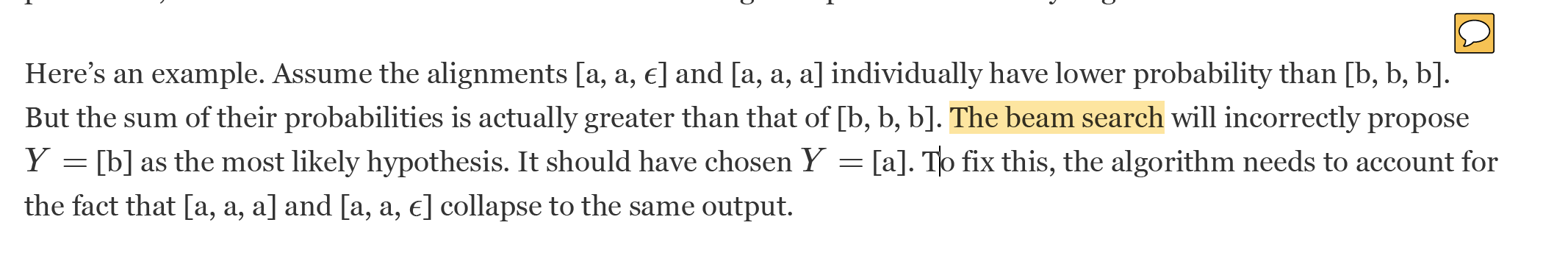This is the first mention of beam search and kind of comes out of no where. Might make sense to make this paragraph after the next one.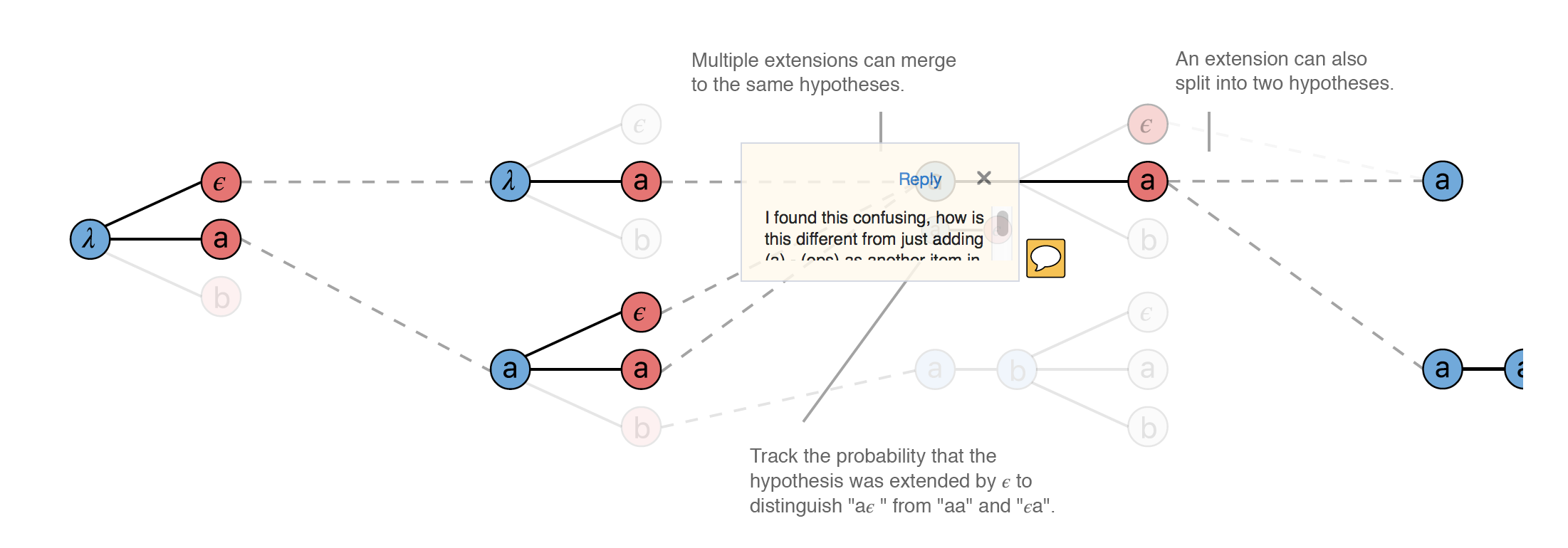I found this confusing, how is this different from just adding (a) - (eps) as another item in the beam? i.e. keeping track of it’s probabilities until it collapses. If it’s not different, it could be helpful to show so in the diagram above (complete beam search) and then use this diagram to show collapsing according to CTC.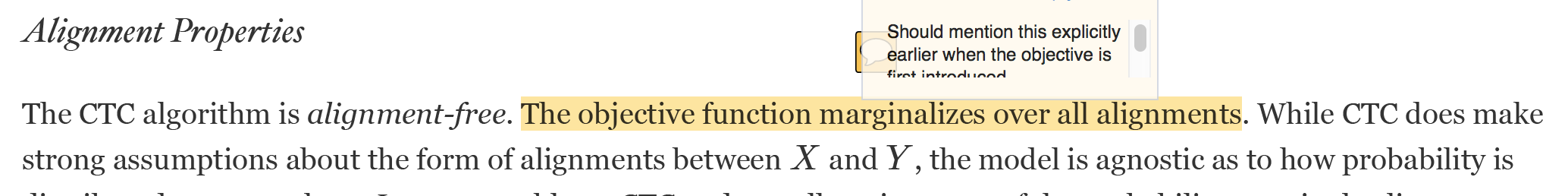Should mention this explicitly earlier when the objective is first introduced.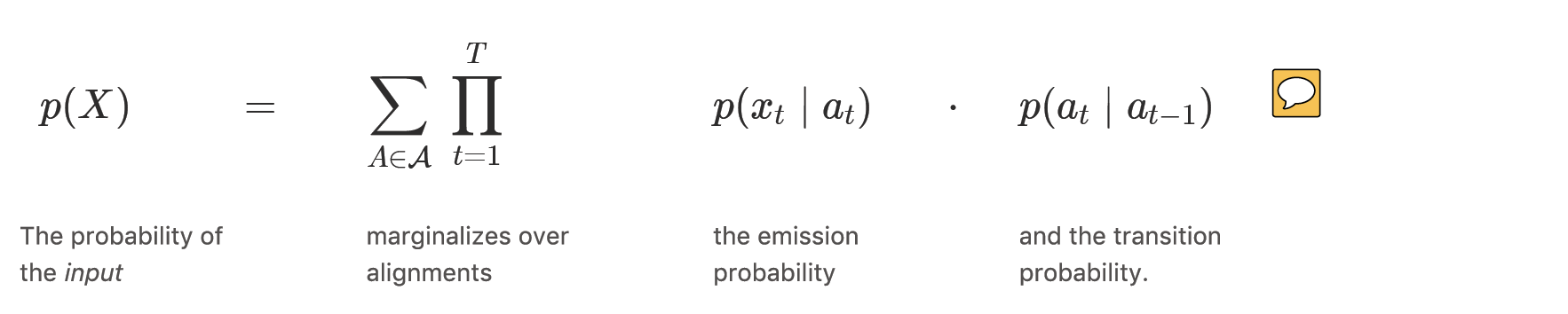Not to be a broken record, but again this section could benefit from clarifying the relationship of p(x|a) and p(x|y)Could benefit from a little more explanation of why you can’t train a model p(x|y) end-to-end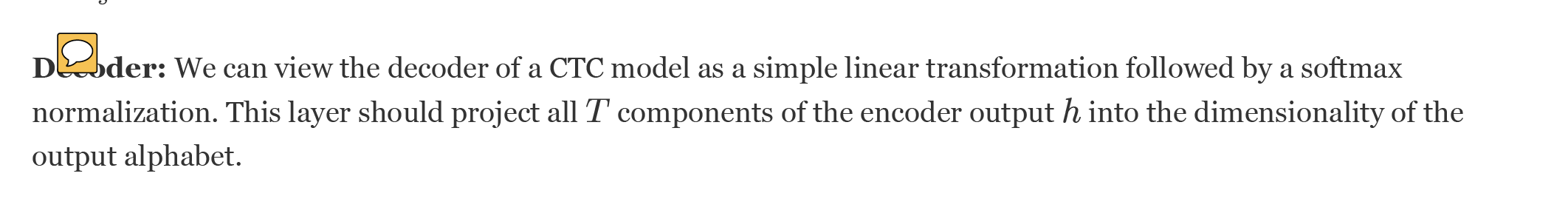Decoders usually incorporate dependence among the outputs y, and attention on h that depends on the outputs. That’s a big adavantage over CTC that is glossed over. The text was updated successfully, but these errors were encountered:
Merged

### awni commented Nov 16, 2017 • edited

 Thanks so much for taking the time to review the article and for the feedback. I've addressed all the points below and the corresponding changes to the article can be found in PR #18 (Report 1). The biggest thing that could use some help is clarifying the formal definition of the alignments A, and how you get p(a|x) i.e. that’s where the function approximator goes. The largest piece feedback from this review is that the article should make more clear where p(a|x) comes from and how it relates to the loss function. I agree that this could be made much better. I've attempted to clarify this in several places. The main changes to address this are: At the top of the alignment section I added a short paragraph motivating and summarizing the relationship of the alignments to the loss function. Included two more steps in the CTC steps figure (first figure in the Loss function section) which shows an example of the input and an RNN which produces the per time-step distribution. I added a paragraph to the loss function section explaining more about how an RNN is usually used to estimate the per time-step distribution. nit: this looks a little like X_s Y_s, maybe a better way of wording this. I converted this to use an apostrophe before the s e.g. X's and Y's. Hopefully that is clear. Maybe clarify here what an alignment means formally. How does p(a|X) relate to p(y|X)? I added a paragraph at the top of the alignment section which motivates the purpose of the alignments and their relationship to the ultimate probability. Based on your feedback, I think this motivation was not clear early on. The CTC algorithm is alignment-free — it doesn't require an alignment between the input and the output. However, to get the probability of an output given an input, CTC works by summing over the probability of all possible alignments between the two. We need to understand what these alignments are in order to understand how the loss function is ultimately calculated. By here it can be extra helpful to clarify where p(a|X) comes from. Maybe talk about p(y|X) coming from a neural network and show audio (X) and P(y|x) as first parts of this diagram?) I've added the network to the figure along with some corresponding text to show where the p(a|X) is coming from. Again, clarifying how to go from p(y|X) to p(z|X) might help. Also, defining the CTC score as it’s relationship to P(Y|X) can be helpful so the reader doesn’t have to wait to find out. I included a line here to make clear that we'’ll use the \alpha's to compute the final CTC score P(Y | X). As to the relationship between p(z|X) and p(y|X): I'm assuming the reviewer meant p_t(y|X) which is the probability of a character at a single time step. This is also what p_t(z|X) is (just using a different variable). Based on changes above, I'm hoping it's sufficiently clear at this point where this per time-step distribution is coming from. The diagrams above were destination centered (arrows bunched by arrows arriving at same destination), but this one is source centered (bunched by leaving from same node), might be clearer to make this destination centered too so you can see the above recursive relations at work. Agreed, this one should also be destination centered also. It has been converted. This is the first mention of beam search and kind of comes out of no where. Might make sense to make this paragraph after the next one. Yes, that should say the "naive heuristic" and not the "beam search" thanks for catching it. The intention of that paragraph is to give an example of the problem with the simple argmax inference algorithm. I found this confusing, how is this different from just adding (a) - (eps) as another item in the beam? i.e. keeping track of it's probabilities until it collapses. If it's not different, it could be helpful to show so in the diagram above (complete beam search) and then use this diagram to show collapsing according to CTC. It's actually different. At time step 3 the beam contains "a" which we can get from the alignments (eps)(a), (a)(a) and (a)(eps). We don't want to treat them individually because we sort the beam based on the probability of “a” not the probability of the individual alignments. The purpose of keeping around the probability of (a)(eps) is that at the next time step (t=4) the "a" can split into both "a" and "aa". The "aa" should only include the probability of (a)(eps)(a) but not the other alignments which would collapse to "a". This is case is definitely the most subtle. I've attempted to explain it clearly in the text between the two figures as well. Should mention this explicitly earlier when the objective is first introduced. This is already mentioned twice in the beginning of the loss function section. Once in the text of first figure which introduces how we arrive at the loss-function from the per time-step probabilities and again in the text when the loss function equation is introduced. Not to be a broken record, but again this section could benefit from clarifying the relationship of p(x|a) and p(x|y) I'm not sure I understand the comment here. The equation pointed out is the relationship between p(X|Y) and the p(x_t|a). This may be a confusion since I dropped the conditioning on Y from the equation to simplify notation. Alternatively, if the reviewer meant p(x_t|y_t) (lower case y as in a member of the sequence Y) - there isn't really a relationship between p(x_t|a) and p(x_t|y) (if Y has the same set of states as A then they're the same thing). (Y is just used here to construct the set of alignments that we are allowed to marginalize over.) Could benefit from a little more explanation of why you can’t train a model p(x|y) end-to-end The term end-to-end is somewhat overloaded / poorly defined and perhaps not the best term to use here. Some comments on this: I think we can certainly model p(x|y) "end-to-end" but for a problem like speech recognition we actually want p(y|x). So modeling p(x|y) is not "end-to-end" in the sense that we're taking a circuitous path towards optimizing p(y|x). So by one interpretation of "end-to-end" just the fact that we’re modeling p(x|y) means we aren’t training a model "end-to-end". At any rate, I think it's best to steer clear of the term "end-to-end" as it's more confusing than illuminating. I've changed the sentence to: Perhaps most importantly, CTC is discriminative. It models p(Y \mid X) directly. This allows us to unleash the capacity of powerful learning algorithms like the recurrent neural network directly towards solving the problem we care about. Decoders usually incorporate dependence among the outputs y, and attention on h that depends on the outputs. That's a big advantage over CTC that is glossed over. My intention for this section was not to compare and contrast the trade-offs of the two models in great detail (which I think is a very interesting question!), but more to develop a common framework for them. However, I did include a short paragraph at the end of this section about the fact that CTC is conditionally independent whereas other encoder-decoders are not but how CTC is still used for tasks like ASR. I think this concludes the section nicely.